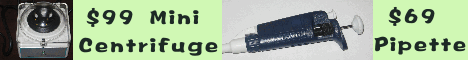The Electronic Protocol Book Table of contents BioToolKit 300 Download Trials An electronic protocol book with 500 protocols and 100 recipes. A great quick and practical reference for bench scientists as well as for new students.   Get A Copy A collection of tools frequently used by bench biomedical scientists, ranging from centrifugation force conversion, molecular weight, OD, recipe calculators, to clinical calculators. Include all Primo 3.4, Abie 3.0, Heatmap Viewer, MicroHelper, Godlist Manager, label printing, and grade book.   More info Log-odds ratio Risk ratio is the ratio of number of subjects with the event in a group to the total in the group. Odds ratio is the ratio of number of subjects with the event in a group to the number of subjects without the event. Log-odds ratio is the natural log of the odds ratio. A risk ratio of 0.2 is equivalent to an odds ratio of 0.25 and a log-odds ratio of -1.4.What is a USB Flash Disk?